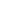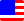Documents  >  Tutorial  >  group2
Operators of the second group

Purpose of this chapter.

We will learn about the dot operator, unary + operator, unary - operator, and logical negation operator.

Operators of the second group.

Operators of the second group are following.

 . x.y dot operator + +x unary + operator - -x unary - operator ! !x logical negation operator

Dot operator

The dot operator is an operation in order to invoke a member of the operand.

This operator call a member of the instance that is returned by the left expression of the operator. The member is specified by the right expression of the operator.

As an example, let's execute a dot operation on an instance of the Circle class.

Circle cir = new Circle(10); // An instance with 10px radius.

The above example instructe to call the Radius getter to the cir variable of the Circle class.

If the left operand is the same as the currently defined class, you can invoke closed members of the class with a dot operation. Otherwise, you can invoke only open members.

If the above example is in the definition of the Circle class, you can invoke closed members of the cir with the dot operator. Because cir is an instance of the Circle class and it is operated within the definition of the Circle class.

This is called class level encapsulation. Rice adopts class level encapsulation.

Unary + operator

The unary + operator returns a new instance with the same value and same sign as the right operand of the operator.

The operand must be the int, long, or real class. If you apply the unary + operator to operand other than these classes, an exception will be thrown.

As an example, let's execute a unary + operation on an instance of the int class.

int i = 10;

int j = i; // i and j refer to same instance.

int k = +i; // +i returns a new instance. Therefore, i and k refer to different instances.

Unary - operator

The unary - operator returns a new instance with the same value and opposite sign as the right operand of the operator.

The operand must be the int, long, or real class. If you apply the unary + operator to operand other than these classes, an exception will be thrown.

As an example, let's execute a unary + operation on an instance of the int class.

int i = 10;

int j = -i; // j is -10.

Logical negation operator

The logical negation operator returns a new instance that inverted the boolean value of the right operand of the operator.

The operand must be the bool class. If you apply the logical negation operator to operand other than bool class, an exception will be thrown.

As an example, let's execute a unary + operation on an instance of the bool class.

bool b = true;

bool c = !b; // c is false.